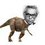# A beautiful equation

Indeed the saying "Curiosity is the mother of inventions" is absolutely true. While learning trigonometry , I found this:

Prove that in $$\Delta ABC$$ ,

$\large\sum_{cyc} \cot A = \prod_{cyc} \csc A + \prod_{cyc} \cot A$

I invite all brilliantians to prove this :)Note by Nihar Mahajan
5 years, 8 months ago

This discussion board is a place to discuss our Daily Challenges and the math and science related to those challenges. Explanations are more than just a solution — they should explain the steps and thinking strategies that you used to obtain the solution. Comments should further the discussion of math and science.

When posting on Brilliant:

• Use the emojis to react to an explanation, whether you're congratulating a job well done , or just really confused .
• Ask specific questions about the challenge or the steps in somebody's explanation. Well-posed questions can add a lot to the discussion, but posting "I don't understand!" doesn't help anyone.
• Try to contribute something new to the discussion, whether it is an extension, generalization or other idea related to the challenge.

MarkdownAppears as
*italics* or _italics_ italics
**bold** or __bold__ bold
- bulleted- list
• bulleted
• list
1. numbered2. list
1. numbered
2. list
Note: you must add a full line of space before and after lists for them to show up correctly
paragraph 1paragraph 2

paragraph 1

paragraph 2

[example link](https://brilliant.org)example link
> This is a quote
This is a quote
    # I indented these lines
# 4 spaces, and now they show
# up as a code block.

print "hello world"
# I indented these lines
# 4 spaces, and now they show
# up as a code block.

print "hello world"
MathAppears as
Remember to wrap math in $$ ... $$ or $ ... $ to ensure proper formatting.
2 \times 3 $2 \times 3$
2^{34} $2^{34}$
a_{i-1} $a_{i-1}$
\frac{2}{3} $\frac{2}{3}$
\sqrt{2} $\sqrt{2}$
\sum_{i=1}^3 $\sum_{i=1}^3$
\sin \theta $\sin \theta$
\boxed{123} $\boxed{123}$

Sort by:

Since, $A+B+C = 180^{o}$

$Cos(A+B+C) = -1$

$\prod_{cyc} \cos (A) - \sum_{cyc} \cos (A) \sin (B) \sin (C) = -1$

Divide both sides with $\prod_{cyc} \sin (A)$,

$\prod_{cyc} \cot (A) - \sum_{cyc} \cot (A) = - \prod_{cyc} \csc (A)$

$\sum_{cyc} \cot (A) = \prod_{cyc} \cot (A) + \prod_{cyc} \csc (A)$

Hence, proved.

- 5 years, 8 months ago

@Nihar Mahajan How is my solution? Is there any other method u r thinking?

- 5 years, 8 months ago

It is quite shorter and elegant than mine.Nice work!

- 5 years, 8 months ago

Wait isn't that what I had told you?

- 5 years, 8 months ago

That day , we both forgot that $\cos(A+B+C)=-1$. Don't worry , it happens sometimes :P

- 5 years, 8 months ago

Do u have another method? Can u please post it?

- 5 years, 8 months ago

I have another method , but it does not use basic results. It uses "lemma type" results , thats because I found this equation while proving that lemma only!

- 5 years, 8 months ago

Please send that solution . I am eager to know another solution to it. Please

- 5 years, 8 months ago

Exactly what is "$cyc$" in $\displaystyle \sum_{cyc}$ and $\displaystyle\prod_{cyc}$? $~~~$ Never seen this.

- 5 years, 8 months ago

$\sum_{cyc} \cot A = \cot A+\cot B+\cot C \\ \prod_{cyc} \cot A = \cot A\cot B\cot C$

It is short form of the cyclic summation/product.I hope you get it now :)

- 5 years, 8 months ago

what does sym summation mean?

- 5 years, 8 months ago

Hello Nihar! Can you please tell me from where are you learning trigonometry?? Thanks 😀

- 5 years, 8 months ago